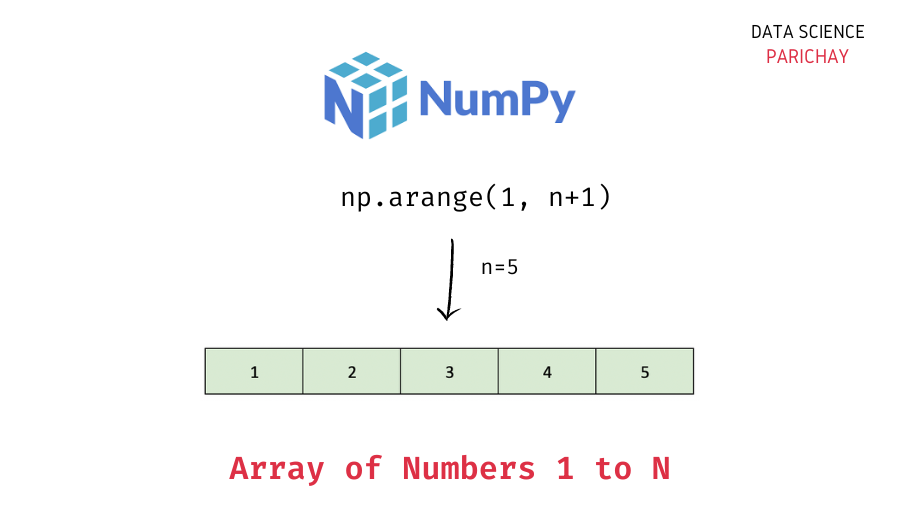# Numpy – Create an Array of Numbers 1 to N

The Numpy library in Python comes with a number of useful functions to create arrays and sequences with custom logic. In this tutorial, we will look at how to create a Numpy array of integers from 1 to N (both included) with the help of some examples.

## How to create an array of integers from 1 to N in Numpy?

You can use the `numpy.arange()` function to create a Numpy array of integers 1 to n. Use the following syntax –

```# create array of numbers 1 to n
numpy.arange(1, n+1)```

The `numpy.arange()` function returns a Numpy array of evenly spaced values and takes three parameters – `start`, `stop`, and `step`.

Values are generated in the half-open interval `[start, stop)` (stop not included) with spacing between the values given by `step` which is `1` by default.

Thus, to get an array of numbers 1 to n, we can use the syntax `numpy.arange(1, n+1)`.

## Examples

Let’s now look at examples of using the above syntax to create an array of integers 1 to n.

### Example 1 – Create a Numpy array of numbers 1 to 5

To create an array of numbers 1 to 5, pass 1 as the start value and 6 (that is, n+1) as the stop value to the `numpy.arange()` function.

```import numpy as np

# create array of numbers 1 to 5 (n=5)
ar = np.arange(1, 6)
# display ar
ar```

Output:

📚 Data Science Programs By Skill Level

Introductory

Intermediate ⭐⭐⭐

🔎 Find Data Science Programs 👨‍💻 111,889 already enrolled

Disclaimer: Data Science Parichay is reader supported. When you purchase a course through a link on this site, we may earn a small commission at no additional cost to you. Earned commissions help support this website and its team of writers.

`array([1, 2, 3, 4, 5])`

We get a 1d array of numbers 1 to 5.

### Example 2 – Create a Numpy array of numbers 1 to 10

Let’s now create an array of numbers 1 to 10 using the `numpy.arange()` function. For this, our start value will be 1 and the stop value will be 11 (that is, n+1).

```# create array of numbers 1 to 10 (n=10)
ar = np.arange(1, 11)
# display ar
ar```

Output:

`array([ 1,  2,  3,  4,  5,  6,  7,  8,  9, 10])`

We get a Numpy array of numbers 1 to 10.

We can use the same template to create an array containing numbers from 1 to any value of n.

## Summary

In this tutorial, we looked at how to use the `numpy.arange()` function to create an array of numbers from 1 to n. An important point to keep in mind is that the `numpy.arange()` function generates evenly spaced values in the half-open interval `[start, stop)` thus, we need to pass `1` as the start value and `n+1` as the stop value –
`numpy.arange(1, n+1)`.

You might also be interested in –

•